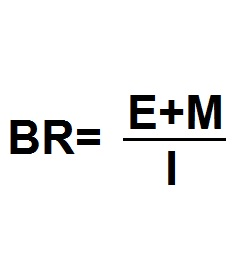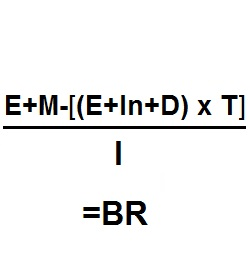Formula - Breakeven Ratio | Propertylogy
Don't Miss

# Formula – Breakeven Ratio

By on August 17, 2018(E+M) / I = BR

Where

BR = Breakeven ratio

E = Operating expenses

M = Mortgage repayment

I = Gross income

The breakeven ratio is a formula used to calculate how sufficient effective gross income is in covering the required cash to run the operations.

When the resulting figure is less than 1, it is generating positive cash flow. And when it’s more than 1, it is cash flow negative.

However, when taxes are required to be accounted for in the calculation, we use the following equation.{E+M-[(E+In+D) x T]} / I = BR

Where

BR = Breakeven ratio net of tax

E = Operating expenses

M = Mortgage repayment

In = Interest

D = Depreciation

I = Gross income

The breakeven ratio net of taxes is a formula that can be very helpful for individual rental property investor when doing tax planning.

You May Also Like...##### How to burn more calories in the office

Send this to a friend